# TensorFlow+TVM优化NMT神经机器翻译

TensorFlow+TVM优化NMT神经机器翻译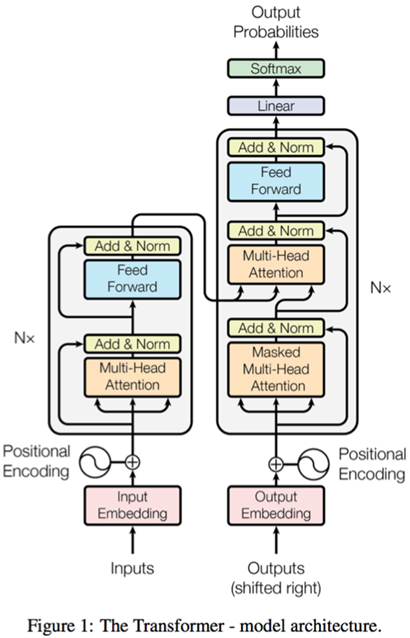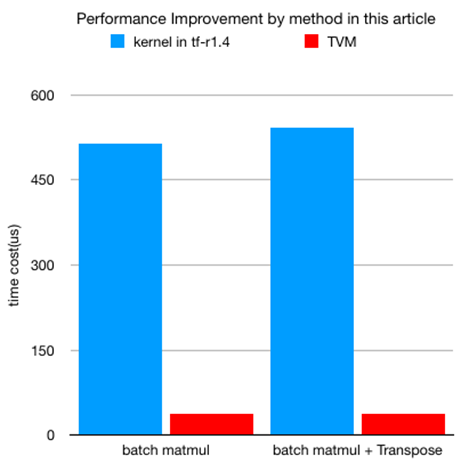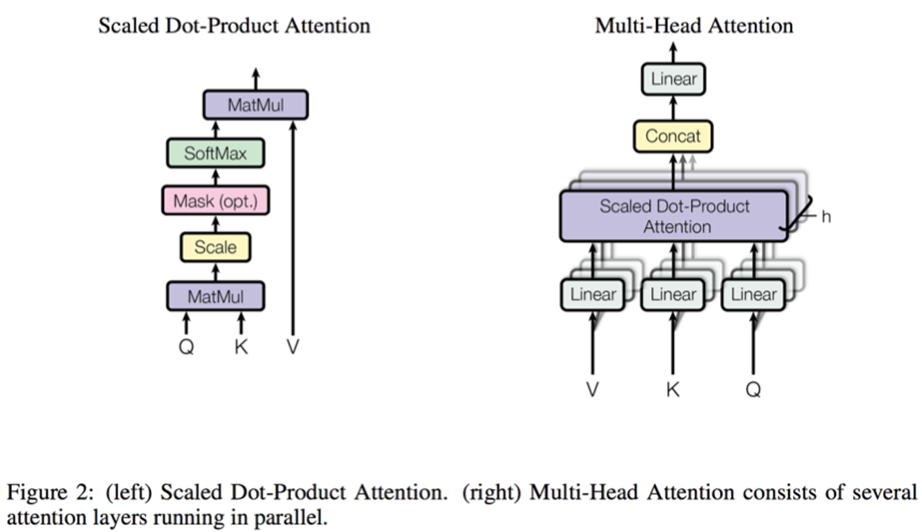void BatchedGemm(input A, input B, output C, M, N, K, batch_dimension) {
for (int i = 0; i < batch_dimension; ++i) {
DoGemm(A[i],B[i],C[i],M,K,N)
}
}

cuBLAS批次batch matmul的性能问题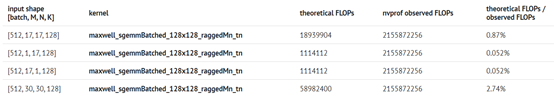cuBLAS的批量matmul实施远非效率。因此，使用TVM为NMT工作负载生成有效的批处理matmul内核。

# computation representation

A = tvm.placeholder((batch, M, K), name=‘A’)
B = tvm.placeholder((batch, K, N), name=‘B’)
k = tvm.reduce_axis((0, K), ‘k’)
C = tvm.compute((batch, M, N),
lambda b, y, x: tvm.sum(A[b, y, k] * B[b, k, x], axis = k),
name = ‘C’)

# thread indices

block_y = tvm.thread_axis(“blockIdx.y”)
block_x = tvm.thread_axis(“blockIdx.x”)
thread_y = tvm.thread_axis((0, num_thread_y), “threadIdx.y”)
thread_x = tvm.thread_axis((0, num_thread_x), “threadIdx.x”)
thread_yz = tvm.thread_axis((0, vthread_y), “vthread”, name=“vy”)
thread_xz = tvm.thread_axis((0, vthread_x), “vthread”, name=“vx”)

# block partitioning

BB, FF, MM, PP = s[C].op.axis
BBFF = s[C].fuse(BB, FF)
MMPP = s[C].fuse(MM, PP)
by, ty_block = s[C].split(BBFF, factor = num_thread_y * vthread_y)
bx, tx_block = s[C].split(MMPP, factor = num_thread_x * vthread_x)
s[C].bind(by, block_y)
s[C].bind(bx, block_x)
vty, ty = s[C].split(ty_block, nparts = vthread_y)
vtx, tx = s[C].split(tx_block, nparts = vthread_x)
s[C].reorder(by, bx, vty, vtx, ty, tx)
s[C].reorder(by, bx, ty, tx)
s[C].bind(ty, thread_y)
s[C].bind(tx, thread_x)
s[C].bind(vty, thread_yz)
s[C].bind(vtx, thread_xz)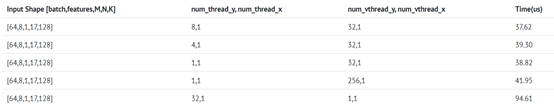# computation representation

A = tvm.placeholder((batch_size, features, M, K), name=‘A’)

# the shape of B is (N, K) other than (K, N) is because B is transposed is this fusion pattern

B = tvm.placeholder((batch_size, features, N, K), name=‘B’)
ENTER = tvm.placeholder((batch_size, 1, M, N), name = ‘ENTER’)
k = tvm.reduce_axis((0, K), ‘k’)
C = tvm.compute(
(batch_size, features, M, N),
lambda yb, yf, m, x: tvm.sum(A[yb, yf, m, k] * B[yb, yf, x, k], axis = k),
name = ‘C’)
D = topi.broadcast_add(C, ENTER)

# computation representation

A = tvm.placeholder((batch_size, features, M, K), name=‘A’)
B = tvm.placeholder((batch_size, features, K, N), name=‘B’)
k = tvm.reduce_axis((0, K), ‘k’)
C = tvm.compute(
(batch_size, M, features, N),
lambda yb, m, yf, x: tvm.sum(A[yb, yf, m, k] * B[yb, yf, k, x], axis = k),
name = ‘C’)

• TF-R1.4 BatchMatmul：513.9
• TF-R1.4 BatchMatmul+ Transpose（另购）：541.9
• TVM BatchMatmul：37.62美元
• TVM BatchMatmul+ Transpose（融合）：38.39美元04-271077
06-154109
11-245
07-022610
01-307296
03-021万+
03-012733
02-025734
07-302867
03-26205
03-26363
03-018833
04-014万+
11-13
08-202639
06-069563
©️2020 CSDN 皮肤主题: 深蓝海洋 设计师:CSDN官方博客